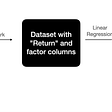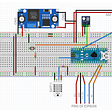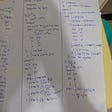# InfluxDB: How to Do Joins, Math across Measurements

1. How to calculate the batch size of lines written to the database per request. Following this example is the fastest way to explore math across measurements. You can simply get the sandbox up and running, and copy and paste the code to try it for yourself.
2. How to “monitor” the efficiency of a heat exchanger over time. You can find the dataset and Flux query for this part are located in this repo.

# How to calculate the batch size of lines written to the database per request

`httpd = from(bucket:"telegraf/autogen")|> range(start: dashboardTime)|> filter(fn:(r) => r._measurement == "influxdb_httpd" and r._field == "writeReq")`
`write = from(bucket:"telegraf/autogen")|> range(start: dashboardTime)|> filter(fn:(r) => r._measurement == "influxdb_write" and r._field == "pointReq")`
`avg_batch_size = join(tables:{httpd:httpd, write:write}, on:["_time"])|> map(fn:(r) => ({_value: r._value_write / r._value_httpd}))|> mean()`

# How to “monitor” the efficiency of a heat exchanger over time

`Th1 = from(bucket: "sensors")  |> range(start: dashboardTime)  |> filter(fn: (r) => r._measurement == "Th1" and r._field == "temperature")  |> drop(columns:["_start", "_stop", "_measurement", "position", “_field”])`
`Th2 = from(bucket: "sensors")  |> range(start: dashboardTime)  |> filter(fn: (r) => r._measurement == "Th2" and r._field == "temperature")  |> drop(columns:["_start", "_stop", "_measurement", "position", “_field”])`
`TH = join(tables: {Th1: Th1, Th2: Th2}, on: ["_time"])`
`TC = join(tables: {Tc1: Tc1, Tc2: Tc2}, on: ["_time"])`
`join(tables: {TC: TC, TH: TH}, on: ["_time"])`
`Th1 = from(bucket: "sensors")  |> range(start: dashboardTime)  |> filter(fn: (r) => r._measurement == "Th1" and  r._field == "temperature")  |> drop(columns:["_start", "_stop", "_measurement", "position", “_field”])   Th2 = from(bucket: "sensors")  |> range(start: dashboardTime)  |> filter(fn: (r) => r._measurement == "Th2" and r. _field == "temperature")  |> drop(columns:["_start", "_stop", "_measurement", "position", “_field”]) TH = join(tables: {Th1: Th1, Th2: Th2}, on: ["_time"])Tc1 = from(bucket: "sensors")  |> range(start: dashboardTime)  |> filter(fn: (r) => r._measurement == "Tc1" and r._field == "temperature")  |> drop(columns:["_start", "_stop", "_measurement", "position", “_field”])  Tc2 = from(bucket: "sensors")  |> range(start: dashboardTime)  |> filter(fn: (r) => r._measurement == "Tc2" and r._field == "temperature")  |> drop(columns:["_start", "_stop", "_measurement", "position", “_field”])   TCTH = join(tables: {Tc1: Tc1, Tc2: Tc2}, on: ["_time"]]) join(tables: {TC: TC, TH: TH}, on: ["_time"])|> map(fn: (r) => (r._value_Tc2 - r._value_Tc1)/(r._value_Th1 - r._value_Th2))|> yield(name: "efficiency")`

--

--

--

## More from Anais Dotis

Love podcasts or audiobooks? Learn on the go with our new app.

## Let’s POP those filters in Tableau!## How to Track Hazardous Asteroids with Composition of Iterators in Python## Difference Between Data Science And Machine Learning With Python?## Overreaction and Underreaction Behavioral Factors## Using Machine Learning To Examine Countries’ Success in the Tokyo 2020 Olympics## Forecasting the Copper Producer Price Index with Prophet## Popular Scraping Libraries## Humidity & Degrees Environment Sensory## Integration## Analysis of Current Anti-Reverse Engineering Techniques